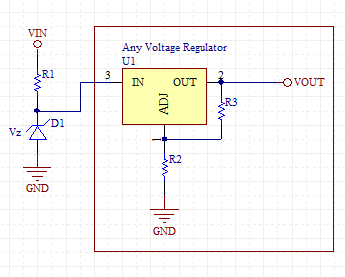# Extending Voltage Input on Voltage Regulators

A common problem with voltage regulators is that the input voltage to the regulator may be higher than the specified maximum input voltage. This schematic shows a very simply way to limit the input voltage to a value that any off the shelf regulator can handle.

The zener diode D1 and Resistor R1 form a crude voltage regulator. The zener voltage should be chosen such that it is less than the maximum input voltage of the regulator. If the input voltage exceeds Vz the zener conducts and the voltage at the input of the regulator is clamped to Vz.

If the voltage is greater than Vz then R1 consumes the following power:

P= (Vin-Vz)^2/R1

If the voltage is less than Vz, then D1 doesn't conduct and R1 wastes some power:

P= R1 * Ireg^2

It's important to minimize R1 such that the voltage across R1 doesn't reduce Vin below the necessary input for the regulator. R1 must be large enough to limit the current to the current rating of the zener. For analysis assume that the regulator draws no current.

R1> (Vin-Vz) / IzenerHigh Input Voltage For Voltage Regulator Schematic

[Plant Database], [Soil Moisture Sensor] [Water Level Sensor] [Soil Moisture Meter]